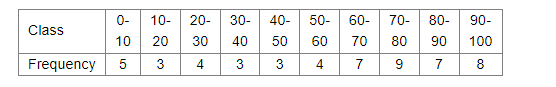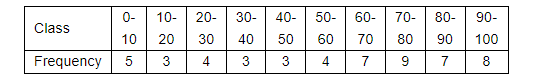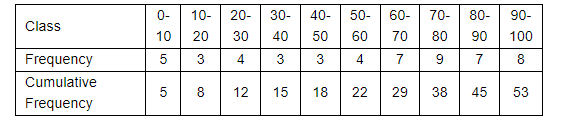# Find the median of the following data`
Question:

Find the median of the following dataSolution:

The given distribution isWe have to find the median of the above distribution.

Now we have to find the cumulative frequency asFrom the above distribution median class is 60-70 and

$l=60$

$N=53$

$h=10$

$f=7$

$C=22$

Now we are using the following relation

median $=l+\frac{\frac{N}{2}-C}{f} \times h$

$=60+\frac{\frac{53}{2}-22}{7} \times 10$

$=66.43$

Hence the median of the given distribution is 66.43